# Test: Application Of Derivative (Competition Level) - 1

## 30 Questions MCQ Test Mathematics (Maths) Class 12 | Test: Application Of Derivative (Competition Level) - 1

Description
This mock test of Test: Application Of Derivative (Competition Level) - 1 for JEE helps you for every JEE entrance exam. This contains 30 Multiple Choice Questions for JEE Test: Application Of Derivative (Competition Level) - 1 (mcq) to study with solutions a complete question bank. The solved questions answers in this Test: Application Of Derivative (Competition Level) - 1 quiz give you a good mix of easy questions and tough questions. JEE students definitely take this Test: Application Of Derivative (Competition Level) - 1 exercise for a better result in the exam. You can find other Test: Application Of Derivative (Competition Level) - 1 extra questions, long questions & short questions for JEE on EduRev as well by searching above.
QUESTION: 1

### The two curves x3 - 3xy2 + 2 = 0 and 3x2 y - y3 - 2 = 0

Solution: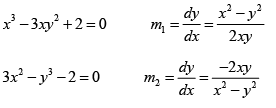m1m2 = -1 ⇒ Curves are orthogonal

QUESTION: 2

### The greatest value of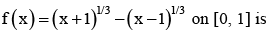Solution: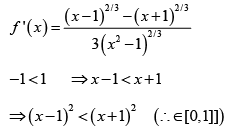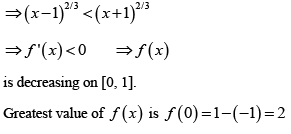QUESTION: 3

### The function f (x) = cot-1 x+ x increases in the interval

Solution: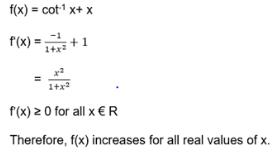QUESTION: 4

The real number x (x > 0) when added to its reciprocal gives the minimum sum at x equals

Solution: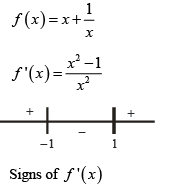Minimum occurs at x = 1

QUESTION: 5

If the function f (x) = 2x3 - 9ax2 + 12a2x + 1, where a > 0 , attains its maximum and minimum at p and q respectively such that p² = q, then ‘a’ equals

Solution: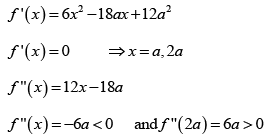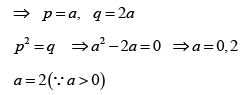QUESTION: 6

If 2a + 3b + 6c = 0, then at least one root of the equation ax2 + bx + c = 0 lies in the interval

Solution: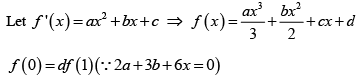By Roll’s theorem, at least one root of f ' (x) =0 lies in (0, 1)

QUESTION: 7

A point on the parabola y2 = 18x at which the ordinate increases at twice the rate of the abscissa, is

Solution:

y² = 18 x       .....(i)
Differentiating w.r.t t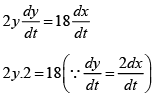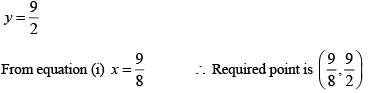QUESTION: 8

The normal to the curve  x = a (1 + cos θ) , y = a sin θ at 'θ' always passes through the fixed point

Solution: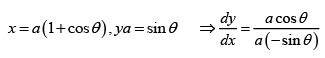Equation of normal at point (a(1 + cos θ) ,a sin θ) is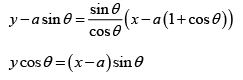It is clear that normal passes through fixed point (a, 0)

QUESTION: 9

Angle between the tangents to the curve y = x2 - 5x + 6 at the points (2, 0) and (3, 0) is

Solution: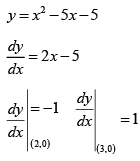Product of slopes = -1 ⇒ angle between tangents is  π/2

QUESTION: 10

A function is matched below against an interval where it is supposed to be increasing, Which of the following pairs is incorrectly matched?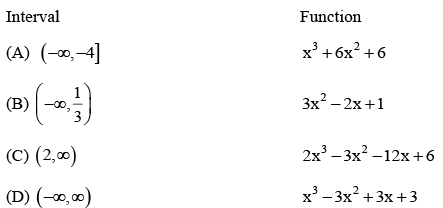Solution: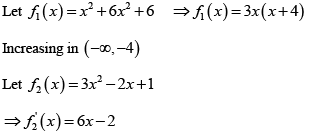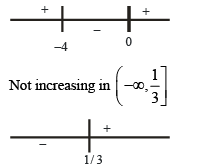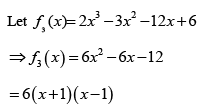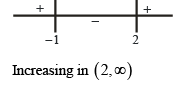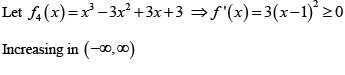QUESTION: 11

A spherical iron ball 10 cm in radius is coated with a layer of ice of uniform thickness that melts at a rate of 50 cm³/min. When the thickness of ice is 15 cm, then the rate at which the thickness of ice decreases, is

Solution: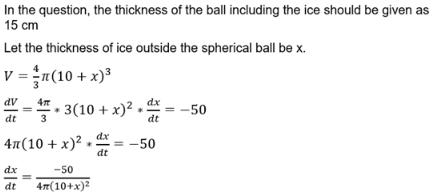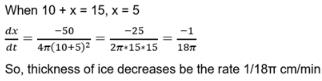QUESTION: 12

Area of the greatest rectangle that can be inscribed in the ellipse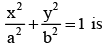Solution:

Any point on ellipse is P (x, y) = (a cosθ, b sinθ)
Area of rectangle = 4ab cosθ sinθ
= 2ab sinθ
∴ Maximum area = 2ab.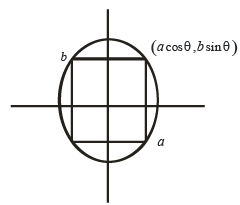QUESTION: 13

Let f (x) be differentiable for all x. If f (1) =-2 and f '(x) > 2 for x ∈ (1, 6) then

Solution: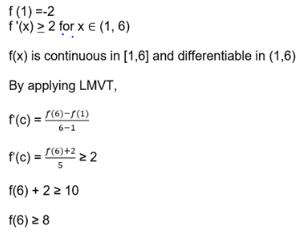QUESTION: 14

The normal to the curve x = a (cos θ + θ sin θ), y = a (sin θ - θ cos θ) at any point 'θ' is such that

Solution: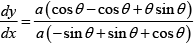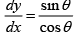Equation of normal is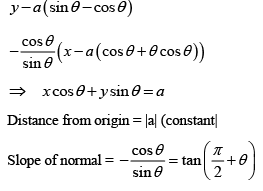⇒ Angle made with x – axis is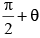QUESTION: 15

The function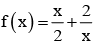has a local minimum at

Solution: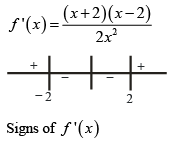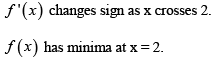QUESTION: 16

A value of c for which the conclusion of Mean value Theorem holds for the function f (x) = loge x on the interval [1, 3] is

Solution: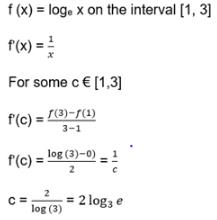QUESTION: 17

The function f (x) =  tan-1 (sin x cos x) is an increasing function in

Solution: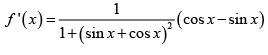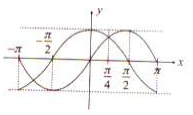QUESTION: 18

Suppose the cubic x3 - px + q = 0 has three distinct real roots where p > 0 and q > 0. Then, which one of the following holds?

Solution:

Graph of y = x2 - px + q cuts x-axis at three distinct pints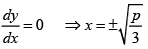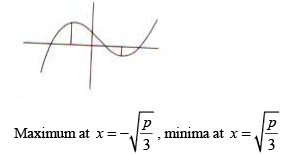QUESTION: 19

The shortest distance between the line y - x= 1 and the curve x =y2 is

Solution:

Condition for shortest distance is slope of tangent to x = y² must be same as slope of line y = x + 1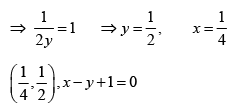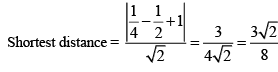QUESTION: 20

Given p (x) = x4 + ax3 + bx2 + cx + d such that x = 0 is the only real root of p' (x) = 0 . If p (-1) < p (1) , then in the interval [-1,1]

Solution: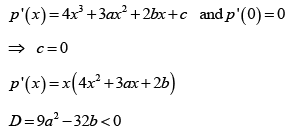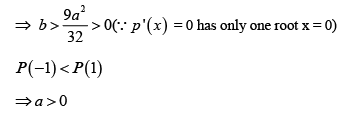p ' (x) has only one change of sign. ⇒ x = 0 is a point of minima.
P(-1) =1- a + b + d P(0) = d
P(1) =1+ a + b + d
⇒ P (-1) < P (1) , P (0) < P (1) , P (-1) > P ( 0)
⇒ P (-1) is not minimum but P(1) is maximum

QUESTION: 21

The equation of the tangent to the curve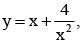that is parallel to the x-axis is

Solution: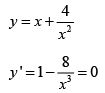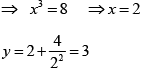(2, 3) is point of contact thus y = 3 is tangent

QUESTION: 22

Let f : R → R be defined by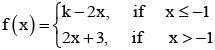If f has a local minimum at x = - 1, then a possible value of k is

Solution: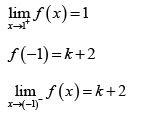∴ f has a local minimum at x = - 1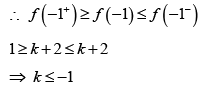Possible value of k is – 1

QUESTION: 23

Let f : R → R be a continuous function defined by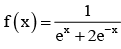Statement-1 :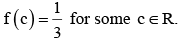Statement -2 :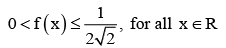Solution: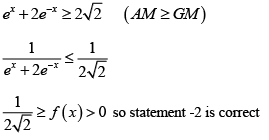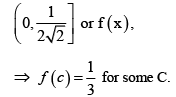Hence correction option is (d)

QUESTION: 24

The normal to the curve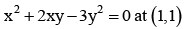Solution: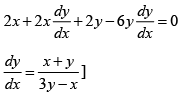Slope of normal at (1, 1) = - 1
∴ Equation of normal y -1 = -1(x -1)
y +x= 2
Solving we get point as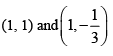QUESTION: 25

Consider the function, f (x) =| x - 2 | + | x - 5 | .x ∈ R
Statement -1 : f '(4) = 0
Statement -2 : f is continous in [2, 5], differentiable in (2, 5) and f (2) = f (5)

Solution:

f (x) is constant is [2, 5]

QUESTION: 26

A spherical balloon is filled with 4500 π cubic meters of helium gas. If a leak in the balloon causes the gas to escape at the rate of 75 π cubic meters per minute, then the rate (in metres per minute) at which the radius of the balloon decreases 49 minutes after the leakage began is

Solution: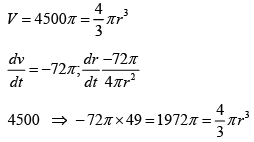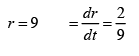QUESTION: 27

Let a, b ∈ R be such that the function f given by f (x) = In | x | +bx2 + ax, x ≠ 0 has extreme values at x =-1 and x = 2.

Statement – 1: f has local maximum at x = - 1 and at x = 2.
Statement-2 :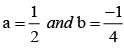Solution: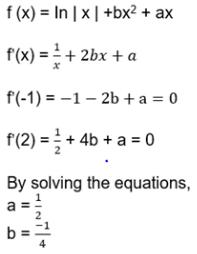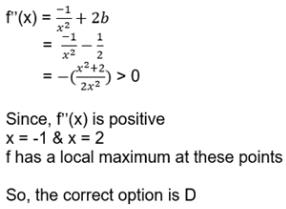QUESTION: 28

The real number k for which the equation, 2x3 + 3x + k = 0 has two distinct real roots in [0, 1]

Solution:

f ' (x) = 6x2+ 3
⇒ so f(x) is increasing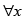equation cannot have 2 distinct roots.

QUESTION: 29

The function f (x) = sin4 x+ cos4 x Increasing if

Solution:

f '(x) = 4 sin3 x cos x - 4 cos3 x sin x = - sin 4 x for increasing f '(x) >0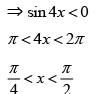QUESTION: 30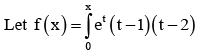dt then f decreasing in the interval

Solution:

f ' (x) < 0
ex (x -1) ( x - 2) < 0
1 < x<2